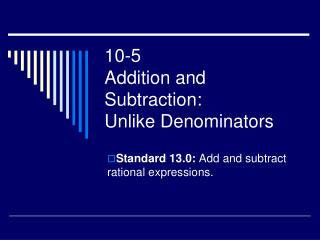DownloadDownload Presentation10-5 Addition and Subtraction: Unlike Denominators

# 10-5 Addition and Subtraction: Unlike Denominators

Télécharger la présentation## 10-5 Addition and Subtraction: Unlike Denominators

- - - - - - - - - - - - - - - - - - - - - - - - - - - E N D - - - - - - - - - - - - - - - - - - - - - - - - - - -
##### Presentation Transcript

1. 10-5Addition and Subtraction:Unlike Denominators Standard 13.0: Add and subtract rational expressions.

2. Factor each expression. Form the product using each factor the greatest number of times it occurs. Least Common Multiple Rule

3. #1 Finding the LCM

4. #2 Finding the LCM

5. Adding and Subtracting Rules: Unlike Denominators • Change Subtraction to Add the Opposite • Factor the Denominator, if possible • Find a Common Denominator (LCD) • Multiply the Numerator and Denominator by the missing factor • Add the Numerator • Factor the Numerator if possible • Simplify if possible

6. Fraction Rule (Addition and Subtraction)

7. #5 Add or subtract. Simplify

8. #6 Add or subtract. Simplify

9. #7 Add or subtract. Simplify

10. #8 Add or subtract. Simplify